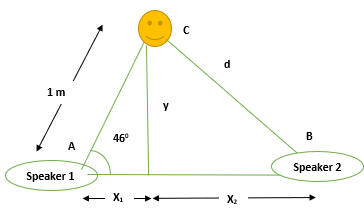# There is a loudspeaker at point A and another point C, where a listener is positioned. |AC| =...

## Question:

There is a loudspeaker at point A and another point C, where a listener is positioned. |AC| = 1.00 m and the angle theta = 46 degrees. A second loudspeaker B is located somewhere to the left of A. The speakers vibrate out of phase and are playing a 66.0 Hz tone. The speed of sound is 343 m/s. What is the second closest distance to speaker A that speaker B can be located, so that the listener hears no sound?

## No sound Effect:

The sound waves from two sound source produce no sound effect for an observer which is positioned at the distance of destructive interference of the waves. The sound amplifies if the position is at the distance of constructive interference.

## Answer and Explanation:

The schematic diagram for the following scenario is given below,The condition for destructive interference is expressed as,

{eq}d_2 - d_1 = \dfrac{n \lambda}{2};\;\;\;\;\;\;n = 1,3,5...\\ \textrm{For minimum distance,} n = 1\\ \therefore d_2 = \dfrac{(1)\lambda}{2}+ d_1\\ \textrm{For} \lambda \textrm{we will use the expression,} v = \lambda f\\ \Rightarrow \lambda = \dfrac{343}{66}\\ \Rightarrow \lambda = 5.19\; \textrm{m}\\ \therefore d_2 = \dfrac{(1)(5.19)}{2}+1 = 3.59\; \textrm{m}\\ {/eq}

For determining the distances, {eq}x_1 \; \textrm{and} \; y {/eq} we will use trigonometric identities and relations,

{eq}x_1 = (1) \cos46° = 0.694\; \textrm{m}\\ y = (1)\sin46° = 0.719\; \textrm{m}\\ \therefore x_2 = \sqrt{d^2_2 + y^2}\\ x_2 = \sqrt{12.92 + 0.482}\\ x_2 = \sqrt{13.402}\\ x_2 = 3.66\; \textrm{m} {/eq}

So, minimum distance comes out to be,

{eq}x_1 +x_2 = 0.694+3.66 = 4.354\; \textrm{m} {/eq}